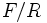# Graph product of groups

This article describes a product notion for groups. See other related product notions for groups.

## Contents

This article is about a definition in group theory that is standard among the group theory community (or sub-community that dabbles in such things) but is not very basic or common for people outside.
VIEW RELATED: Analogues of this | Variations of this | Opposites of this |
View a list of other standard non-basic definitions

## Definition

Let$X = (V,E)$ be a graph. To each$v$ in$V$, associate a vertex group$G_v$. Then, the graph product of the$G_v$s with respect to the graph$X$ is defined as$F/R$ where$F$ is the free product of all the$G_v$s and$R$ is the normal subgroup generated by subgroups of the form$[G_u,G_v]$ whenever there is an edge joining$u$ and$v$.

## Particular cases

### Direct product

The external direct product (restricted version) of a family of groups is precisely the same as the graph product with these as the vertex groups and the graph as the clique.

### Free product

The free product of a family of groups is precisely the same as the graph product with these as the vertex groups and the graph taken as the empty graph.